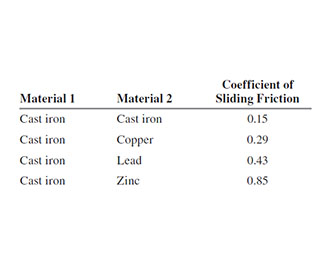# Problem: A long ramp made of cast iron is sloped at a constant angle kg = 52.0m/s above the horizontal. Small blocks, each with mass 0.42 m kg but made of different materials, are released from rest at a vertical height h above the bottom of the ramp. In each case the coefficient of static friction is small enough that the blocks start to slide down the ramp as soon as they are released. You are asked to find h so that each block will have a speed of 4.00 m m/s when it reaches the bottom of the ramp. You are given these coefficients of sliding (kinetic) friction for different pairs of materials .Use work and energy considerations to find the required value of h if the block is made from cast iron.Use work and energy considerations to find the required value of h if the block is made from copper.Use work and energy considerations to find the required value of h if the block is made from zinc.What is the required value of h for the copper block if its mass is doubled to 0.84 kg?For a given block, if heta is increased while h is kept the same, does the speed v of the block at the bottom of the ramp increase, decrease, or stay the same?

⚠️Our tutors found the solution shown to be helpful for the problem you're searching for. We don't have the exact solution yet.

###### Problem Details

A long ramp made of cast iron is sloped at a constant angle kg = 52.0m/s above the horizontal. Small blocks, each with mass 0.42 but made of different materials, are released from rest at a vertical height h above the bottom of the ramp. In each case the coefficient of static friction is small enough that the blocks start to slide down the ramp as soon as they are released. You are asked to find h so that each block will have a speed of 4.00 when it reaches the bottom of the ramp. You are given these coefficients of sliding (kinetic) friction for different pairs of materials.

Use work and energy considerations to find the required value of h if the block is made from cast iron.

Use work and energy considerations to find the required value of h if the block is made from copper.

Use work and energy considerations to find the required value of h if the block is made from zinc.

What is the required value of h for the copper block if its mass is doubled to 0.84 kg?

For a given block, if is increased while is kept the same, does the speed of the block at the bottom of the ramp increase, decrease, or stay the same?